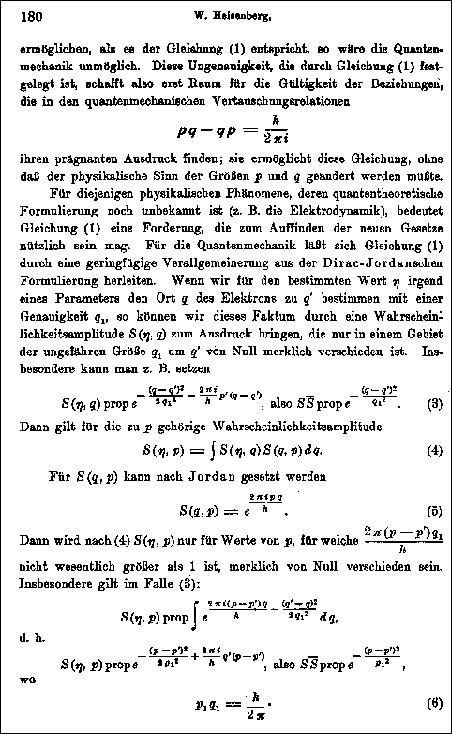Family Matters

1901-1910

High School Student

1911-1920

Youth Movement

1911-1920

University Student

1920-1927

Heisenberg's Doctorate

1920-1927

The Quantum Mechanic

1925-1927

The Uncertainty Principle

1925-1927

The Copenhagen Interpretation

1925-1927

Professor in Leipzig

1927-1942

Fission Research

1939-1945

Reviving German Science

1946-1976

Physics and Philosophy

1955-1956

A Brief Chronology

1901-1976

# Uncertainty RelationsThis excerpt from Heisenberg's original paper shows his derivation of the uncertainty relation for momentum and position.

Here Heisenberg transforms the "bell curve" in quantum theory for the imprecision in the measurement of the position q of a moving electron to the corresponding bell curve of the measured momentum p. The uncertainty, or standard deviation, of each curve is Dp=p1 and Dq=q1. Heisenberg obtains an equal sign for the minimum uncertainty. He later included the possibility of even greater uncertainty by using the sign for "greater than or equal to."

Reference: Zeitschrift für Physik, 43 (1927), 172-198, received 23 March 1927. The image is from the facsimile reprint in Heisenberg, Gesammelte Werke / Collected Works, vol. A1, p. 486.

Heisenberg's uncertainty paper has been translated into English by John Archibald Wheeler and Hubert Zurek, in Quantum Theory and Measurement, Wheeler and Zurek, eds. (Princeton: Princeton Univ. Press, 1983), pp. 62-84.

Following Heisenberg's derivation of the uncertainty relations, one starts with an electron moving all by itself through empty space. To describe the electron, a physicist would refer to certain measured properties of the particle. Four of these measured properties are important for the uncertainty principle. They are the position of the electron, its momentum (which is the electron's mass times its velocity), its energy, and the time. These properties appear as "variables" in equations that describe the electron's motion.

The uncertainty relations have to do with the measurement of these four properties; in particular, they have to do with the precision with which these properties can be measured. Up until the advent of quantum mechanics, everyone thought that the precision of any measurement was limited only by the accuracy of the instruments the experimenter used. Heisenberg showed that no matter how accurate the instruments used, quantum mechanics limits the precision when two properties are measured at the same time. These are not just any two properties but two that are represented by variables that have a special relationship in the equations. The technical term is "canonically conjugate" variables. For the moving electron, the canonically conjugate variables are in two pairs: momentum and position are one pair, and energy and time are another. Roughly speaking, the relation between momentum and position is like the relation between energy and time.

The uncertainty relations involve the uncertainties in the measurements of these variables. The "uncertainty" -- sometimes called the "imprecision"--is related to the range of the results of repeated measurements taken for a given variable. For example, suppose you measure the length of a book with a meter stick. It turns out to be 23.6 cm, or 23 centimeters and 6 millimeters. But since the meter stick measures only to a maximum precision of 1 mm, another measurement of the book might yield 23.7cm or 23.5 cm. In fact, if you perform the measurement many times, you will get a "bell curve" of measurements centered on an average value, say 23.6 cm. The spread of the bell curve, or the "standard deviation," will be about 1 mm on each side of the average. This means that the "uncertainty" or the precision of the measurement is plus or minus 1 mm.

The uncertainty relations may be expressed in words as follows.

#### The simultaneous measurement of two conjugate variables (such as the momentum and position or the energy and time for a moving particle) entails a limitation on the precision (standard deviation) of each measurement. Namely: the more precise the measurement of position, the more imprecise the measurement of momentum, and vice versa. In the most extreme case, absolute precision of one variable would entail absolute imprecision regarding the other.

The uncertainty relations can be written a little more precisely in the shorthand of mathematical symbols. But first we must define what these symbols stand for. Here are their definitions: Dq is the uncertainty or imprecision (standard deviation) of the position measurement.

Dp is the uncertainty of the momentum measurement in the q direction at the same time as the q measurement.

DE is the uncertainty in the energy measurement.

Dt is the uncertainty in the time measurement at the same time as the energy is measured.

h is a constant from quantum theory known as Planck's constant, a very tiny number.

p is pi from the geometry of circles.

means "greater than or equal to"

Putting these symbols together, the two uncertainty relations look like this:

### DE Dt > h / 4p

Let's say you measure the position of a moving electron with such great accuracy that Dq is very small. What happens to the precision of the momentum Dp, which you measure at the same instant? From the first relation, we have

### Dp > h / 4 p Dq

You can see that the uncertainty in the momentum measurement, Dp is very large, since Dq in the denominator is very small. In fact, if the precision of the position measurement gets so great that the uncertainty Dq gets so small that it approaches zero, then Dp gets so large that it approaches infinity or becomes completely undefined.

To see if the uncertainty relations are more than just a mathematical result, Heisenberg considered a thought experiment. From this thought experiment, and another for energy and time, Heisenberg concluded that the mutual uncertainties in position and momentum or energy and time really do exist. They are not the fault of the experimenter or the apparatus. They are a fundamental consequence of the quantum equations, built into every experiment in which quantum mechanics comes into play.